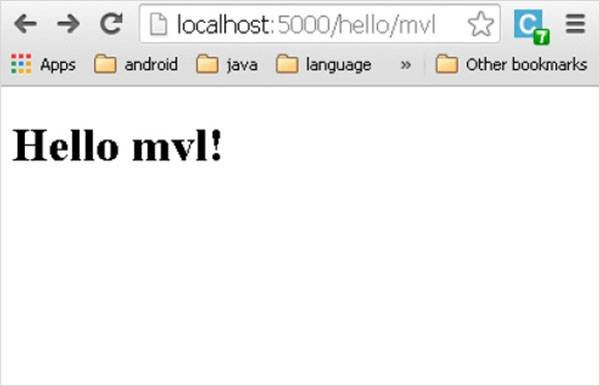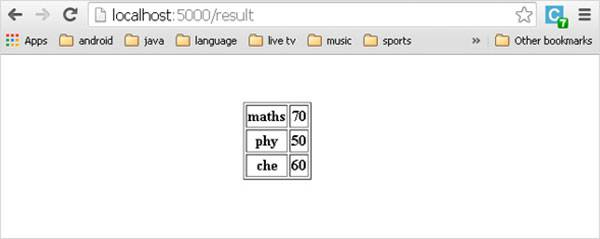# 标签呈现'Hello World'。

```from flask import Flask
@app.route('/')
def index():
return ''Hello World''
if name == 'main':
app.run(debug = True)
```

```from flask import Flask，render_template
@app.route('/')
def index():
return render_template(‘hello.html’)
if name == 'main':
app.run(debug = True)
```

• Application folder
• Hello.py
• templates
• hello.html

```<!doctype html>

```  <h1>Hello {{ name }}!</h1>
```   ```

```from flask import Flask, render_template
@app.route('/hello/')
def hello_name(user):
return render_template('hello.html', name = user)
if name == 'main':
app.run(debug = True)
```

URL的变量部分插入{{name}}占位符。Jinja2模板引擎使用以下分隔符从HTML转义。

• {% ... %}用于语句
• {{ ... }}用于表达式可以打印到模板输出
• {# ... #}用于未包含在模板输出中的注释
• # ... ##用于行语句

Python脚本如下：

```from flask import Flask, render_template
@app.route('/hello/')
def hello_name(score):
return render_template('hello.html', marks = score)
if name == 'main':
app.run(debug = True)
```

hello.html的HTML模板脚本如下：

```<!doctype html>

```  {% if marks>50 %}
{% else %}
{% endif %}
```   ```

Python循环结构也可以在模板中使用。在以下脚本中，当在浏览器中打开URL http://localhost:5000/result时，result()函数会将字典对象发送到模板results.html

result.html的Template部分使用for循环将字典对象result{}的键和值对呈现为HTML表的单元格。

```from flask import Flask, render_template
@app.route('/result')
def result():
dict = {'phy':50,'che':60,'maths':70}
return render_template('result.html', result = dict)
if name == 'main':
app.run(debug = True)
```

```<!doctype html>

```  <table border = 1>
{% for key, value in result.iteritems() %}
&lt;tr&gt;
&lt;th&gt; {{ key }} &lt;/th&gt;
&lt;td&gt; {{ value }} &lt;/td&gt;
&lt;/tr&gt;

{% endfor %}
``````  </table>
```   ```App下载